### Home > CALC > Chapter Ch3 > Lesson 3.3.2 > Problem3-102

3-102.
1. Find f ′(x) for each of the following functions. Homework Help ✎

1.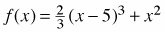2.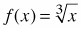3.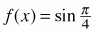4.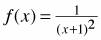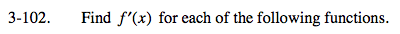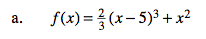f '(x) = 2(x − 5)2 + 2xBefore you find the derivative, rename f(x) using laws of exponent.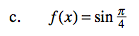$\text{ Careful. What is sin}\frac{\pi }{4}?$

$\text{ Describe the graph of }y=\text{ sin}\frac{\pi }{4}.$

Describe the behavior of its slope.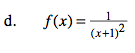Refer the the hint in part (b).